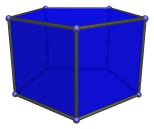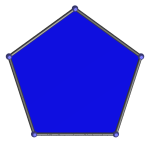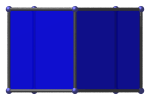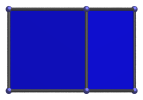# The Pentagonal Prism

The pentagonal prism is a 3D uniform polyhedron bounded by 7 polygons (2 pentagons and 5 squares), 15 edges, and 10 vertices. It may be considered to be the extrusion of the pentagon.The pentagonal prism can be augmented by a square pyramid to form the augmented pentagonal prism (J52), a Johnson solid. A second square pyramid can be added at a non-adjacent position to produce the biaugmented pentagonal prism (J53).

## Projections

In order to be able to identify the pentagonal prism in various projections of 4D objects, it is useful to know how it appears from various viewpoints. The following are some of the commonly-encountered views:

Projection Envelope DescriptionRegular pentagon

Parallel projection centered on a pentagonal face.Rectangle

Parallel projection centered on a vertical edge.Rectangle

Parallel projection parallel to a square face. The left edge of the projection envelope is the image of a square face.

## Coordinates

The Cartesian coordinates of the pentagonal prism, centered on the origin and having edge length 2, are:

• (√((10+2√5)/5), 0, ±1)
• (√((5−√5)/10), ±φ, ±1)
• (−√((5+2√5)/5), ±1, ±1)

where φ=(1+√5)/2 is the Golden Ratio.

## Occurrences

The pentagonal prism occurs in the following 4D uniform polytopes:

The pentagonal prism also occurs in the following CRF polychora:

Last updated 17 Jun 2019.